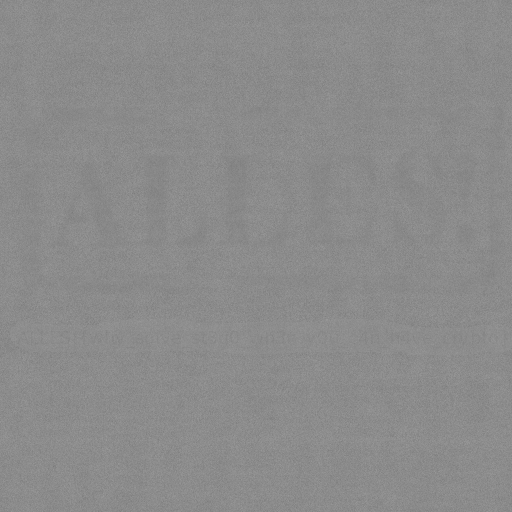# ALLES CTF 2021: Nostego

## September 05, 2021

Reading time ~2 minutes

Unfortunately infrastructure problems meant this CTF was quite frustrating to play. I spent most of my time refreshing a website waiting for my session to connect so I only solved two challenges before moving on to GrabCON. Anyway I solved Nostego first so here’s my solution. I believe my solution is pretty wrong but it worked so /shrug.

#### Nostego - Crypto - 129 points

``````It cannot be stego because the source is attached.

Challenge Files:ALLES.enc.png challenge.py

(100 solves)
``````

With the challenge we get this file:

• `ALLES.enc.png`
• `challenge.py`

The Python script is the method used to “encrypt” the flag which is in a PNG file originally:

``````from PIL import Image
import sys

if len(sys.argv) != 3:
print("Usage: %s [infile] [outfile]" % sys.argv)
sys.exit(1)

image = Image.open(sys.argv).convert("F")
width, height = image.size
result = Image.new("F", (width, height))

ROUNDS = 32

for i in range(width):
for j in range(height):
value = 0
di, dj = 1337, 42
for k in range(ROUNDS):
di, dj = (di * di + dj) % width, (dj * dj + di) % height
value += image.getpixel(((i + di) % width, (j + dj + (i + di)//width) % height))
result.putpixel((i, j), value / ROUNDS)

result = result.convert("RGB")
result.save(sys.argv)
``````

I wasn’t very sure how to solve it properly but I had one idea. Since the starting values of `di` and `dj` are known and they only change modulo the PNG width and height we know what each value was over time.

My idea was to replay the same transforms on the file backwards and see what happens.

So firstly I recorded all of the `di, dj` values running forward:

``````from PIL import Image
import sys

if len(sys.argv) != 3:
print("Usage: %s [infile] [outfile]" % sys.argv)
sys.exit(1)

image = Image.open(sys.argv).convert("F")
width, height = image.size
result = Image.new("F", (width, height))

ROUNDS = 32

f = open('tuples.csv', 'w')
for i in range(width):
for j in range(height):
value = 0
di, dj = 1337, 42
for k in range(ROUNDS):
di, dj = (di * di + dj) % width, (dj * dj + di) % height
f.write('%d,%d\n' % (di,dj))
value += image.getpixel(((i + di) % width, (j + dj + (i + di)//width) % height))
result.putpixel((i, j), value / ROUNDS)

f.close()
result = result.convert("RGB")
result.save(sys.argv)
``````

Then I replayed them in reverse to the encrypted file:

``````from PIL import Image

image = Image.open("ALLES.enc.png").convert("F")
width, height = image.size
result = Image.new("F", (width, height))

ROUNDS = 32

lines = iter(open('tuples.csv', 'r').readlines()[::-1])
for i in range(width):
for j in range(height):
value = 0
for k in range(ROUNDS):
line = next(lines)
di = int(line.split(","))
dj = int(line.split(","))
value += image.getpixel(((i - di) % width, (j - dj + (i - di)//width) % height))
result.putpixel((i, j), value / ROUNDS)

result = result.convert("RGB")
result.show()
result.save("out.png")
``````

Which gives me this image:Which you can barely see the outline of the ALLES! CTF logo. Underneath that its also possible to make out some of the flag. I used `gimp` to make the flag more visible using `sharpen` and this is what I got:Which isn’t a great solution I agree but with some squinting I was able to make out the flag:

`ALLES!{why_so1v3_st3g0_wh3n_y0u_c4n_h4v3_crypto}`

It took a few guesses about some of the characters but it sure beat spending a lot more time on a perfect solution :)

### niteCTF - CBC-Jail

A unique combination of Python jailbreak and crypto flaw that had me learning a lot about AES-CBC mode. Super fun for me to get this solu...… Continue reading

#### niteCTF - Rabin to the Rescue

Published on December 12, 2021

#### HTB CyberSanta 2021 - Crypto Writeups

Published on December 04, 2021